# (Back to) Square One / Cube 21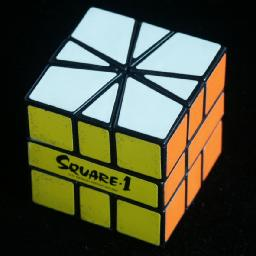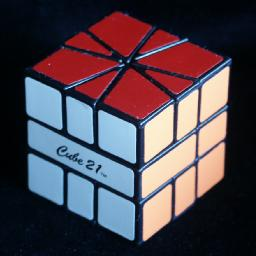Introduction:
1. Description
2. The number of positions
Proof of positions counting formula
3. God's Algorithm
Full God's Algorithm results
4. Programs

Solution:
1. Getting into cube shape
Diagram of shapes
Method 1: Gathering corners
Method 2
Method 3: Pairing the edges
2. Solving the pieces
Notation
A short note about odd permutations
Method 1 Separate layers, then solve corners, then edges.
Phase 1: Put pieces in their layers.
Phase 2: Put the corners correct.
Phase 3: Put the edges correct.
Method 2 Solve corners, then edges.
Phase 1: The corner pieces.
Phase 2: Place the edge pieces in their correct layer.
Phase 3: Place the edge pieces in each layer correctly.
Phase 4: Fix the central layer.
Method 3 Solve edges, then corners.
Method 4 Separate layers, then solve each layer.
Phase 1: Put pieces in their layers.
Phase 2: Solve each layer separately.
Method 5 Separate layers, solve parity, then each layer.
Phase 1: Put pieces in their layers.
Phase 2: Fix parities.
Phase 3: Solve first layer.
Phase 4: Solve second layer.
Method 6 Pair up corners/edges, then solve as domino.
Phase 1: Pair up corners and edges
Phase 2: Solve as Domino corners
Odds and Ends

## Description:

This puzzle is a cube consisting of three layers. The top and bottom layers are cut like a pie in 8 pieces; 4 edge pieces and 4 corner pieces, 30 and 60 degrees wide respectively. The top and bottom layers can rotate. The middle layer is cut in only two halves along one of the lines of the other layers. If there are no corner pieces in the way, you can twist half the cube 180 degrees so that pieces from the top and bottom layers mingle.

The puzzle is unique in that the two types of pieces intermingle. The edge and corner pieces can freely move between the two outer layers. Of course, the puzzle will not necessarily be a cube shape if the pieces are mixed. The puzzle has six colours, each face has a single colour similar to the Rubik's cube.

Square-1 was patented by Karel Hrsel and Vojtech Kopsky on 16 March 1993, US 5,193,809.

## The number of positions:

There are three categories of puzzle shapes.
1. Both layers have 4 edges and 4 corners each.
2. One layer has 3 corners, 6 edges, the other 5 corners 2 edges.
3. One layer has 2 corners, 8 edges, the other 6 corners and no edges.

There are 1, 3, 10, 10 and 5 layer shapes with 6, 5, 4, 3 and 2 corners. This means there are 5·1+10·3+10·10+3·10+1·5 = 170 shapes for the top and bottom layers. The middle layer has two shapes (half of it is assumed to be fixed). This means that there seem to be 170·2·8!·8! = 552,738,816,000 positions if we disregard rotations of the layers. Some layer shapes however have symmetry, and these have been counted too many times this way.

To take account of the symmetries we can simply count the number of layer shapes differently. Instead of the numbers 1, 3, 10, 10, 5 we use the numbers 2, 36, 105, 112, 54, which are the number of shapes if we consider rotations different (e.g. a square counts as 3 because it has three possible orientations). By the same method as before we then get 19305·2·8!·8! or 62,768,369,664,000 positions. To exclude layer rotations, divide by 122 to get a total of 435,891,456,000 distinct positions.

It is interesting to note that this number is exactly 15!/3. In fact, even for the generalised version with 2n pieces in each layer, a similarly simple formula holds, viz. 4(4n-1)!/3n. I had proved this in a rather complicated unsatisfactory way, but Mike Godfrey found a neat insightful proof. He even generalised it to 8(C+E-1)!/(2C+E), where C and E are the total number of corner and edge pieces, and E>0.

If instead we wish to count only all those positions where there are no corner pieces in the way of twisting the halves, then we can use the same method but counting only all the different ways each shape can be split into two halves, e.g. a square counts as 2 this time. The numbers to plug in are now 1, 12, 46, 62, and 37 which gives a total of 3678·2·8!·8! = 11,958,666,854,400 twistable positions.

## God's Algorithm:

In 2005 Mike Masonjones calculated God's Algorithm for the Square-1 in the twist metric. This shows that every position can be solved in at most 13 twists (10.615 on average) or 12 if the middle layer is ignored. The full results for each shape are on a separate page, but the totals can be seen in the table below.

In 2017 Shuang Chen calculated God's Algorithm for the Square-1 in the face turn metric. Every position can be solved in at most 31 face turns (25.134 on average).
TwistsPositions
01
164
21153
317050
4235144
53091458
638893230
7452031138
84459167504
933671064770
10149502310936
11183662070768
1263945120032
13157452752
Total:435891456000
Face turnsPositions
0 1
1 15
2 69
3 212
4 1,141
5 3,933
6 14,029
7 44,188
8 138,952
9 446,720
10 1,316,172
11 4,175,446
12 12,147,472
13 37,884,628
14 109,466,234
15 332,879,292
Face turnsPositions
16 956,379,612
17 2,830,921,668
18 8,002,926,230
19 22,662,798,296
20 61,124,357,782
21 160,994,777,902
22 396,626,886,108
23 927,646,447,818
241,889,794,966,390
253,128,948,769,310
263,301,932,153,566
271,766,634,454,944
28 281,534,253,876
29 8,469,254,908
30 8,987,110
31 376
Total:11,958,666,854,400

## Programs:

To help solve this puzzle I have written computer programs that search for solutions for a particular position. The first Square-1 Solver is similar in style to Kociemba's algorithm for the Rubik's cube, as it solves it in two stages. In the first stage it makes a cube with an even permutation of pieces, and the second stage solves it. With some simple look-up tables the search is speeded up. This program has helped me improve some of the move sequences in my previous solution method, and helped me find many others.
If you have more than 75 Megabytes of Ram, then you are better off with my second program, the Square-1 optimiser. It searches for optimal solutions only, using a single phase search with larger pruning tables. This fast program helped me turn all the sequences on this page into optimal ones.
From the results of the two programs I suspect that God's algorithm uses at most about 30 moves in total. Mike Godfrey has optimally solved over 300,000 positions, and found two positions at depth 30.

## Section 1:

Getting the cube shape.

First you must get the puzzle into cube shape. This takes at most 7 twists (ignoring the centre layer shape), and the shortest method is simply using a table to look up the moves since there are only 90 different possible puzzle shapes. Such tables can be found at various websites, but they are not particularly enlightening. Instead I will present three methods here. The first is the most intuitive, and is the most common way of doing this phase. The second method is very different, but interesting as it shows an underlying mathematical constraint that I find quite surprising. The third is a little similar to the first though described in much more detail.

I have made a diagram showing the various shapes. At the top is the solved cube shape, and each successive row below it is one move further away. The blue shapes have 6 corners in one layer and two in the other, and these shapes are used in method 1 below. The red shapes have five and three corners in the layers. The yellow, green and purple ones have 4 corners in each layer, and the three colours signify the type of shape as used in method 2.

Method 1: Gathering corners

This method simply involves bringing six of the corners into one layer, which leaves the remaining pieces (2 corners and 8 edges) in the other layer. From such a position it is fairly easy to recover the cube shape.

I will not go into detail on bringing 6 corners together as it is quite easy. Once you have three corners together in half of one layer, then move these pieces around to the left half of the bottom layer. Now keep them there, so only use twists of the right hand side and turns of the top layer, and try to bring three more corners together in the top layer. Finally bring these two sets of three together in one layer.

Hold the puzzle with the six corners in the bottom layer. The top layer has two corners and six edges. There are only 5 possible shapes it can have, because there must be anywhere from 0 to 4 edge pieces between the two corner pieces. The diagrams below show how the cube shape can be recovered in each case.

 0 /(-2,-4)/(-1,-2)/(-3,-3)/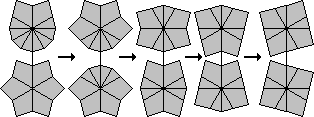1 /(2,-2)/(-3,-4)/(4,-3)/(-5,-4)/(6,-3)/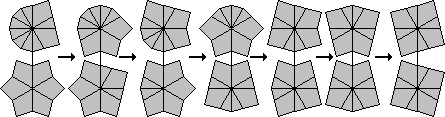2 /(-4,-2)/(-1,4)/(-3,0)/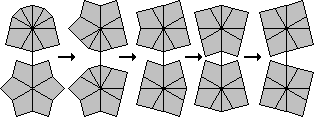3 /(-4,0)/(5,4)/(2,-3)/(-5,-4)/(6,-3)/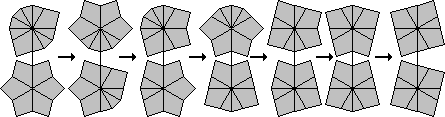4 /(2,2)/(0,-1)/(3,3)/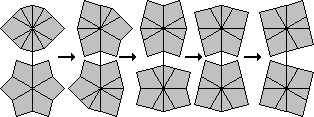The notation used here will be explained later when it will be more extensively used instead of diagrams.

Method 2:
This is a method found in the rec.puzzles archive.
There are 10 shapes with 4 edges (e), 4 corners (c) that a layer can have. Looking clockwise around the layer, they are: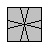cececece: Square 0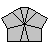ccecceee: Mushroom 0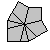ccceecee: Shield 0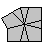cceecece: Right Fist 1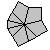ccceceee: Left Paw 1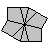cceeccee: Barrel 2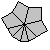cccceeee: Scallop 2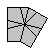cceceece: Kite 2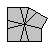ccececee: Left Fist 3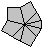ccceeece: Right Paw 3

If you are in a position with two of these shapes, look up the numbers associated with them in the list above and add them to get a number. Whatever move you do that keeps 4 corners in each layer, the number arrived at will not change (modulo 4). In particular if the number is not 0 (or 4) you cannot get it into a cube shape without first moving to a position that does not have 4 corners on each side. You then have to move back to a position with 4 corners in each layer and that does have a 0 or 4 sum.

If you do get a 0 (or 4) sum then the following list shows you how far away you are from the cube shape:

 Square-Square 0 Kite-Kite 1
 Barrel-Barrel 2 Shield-Shield 3 Scallop-Scallop 3 Mushroom-Mushroom 3 Barrel-Scallop 4
 L.Fist-R.Fist 2 L.Paw-R.Paw 3 Barrel-Kite 3 Square-Shield 3 Square-Mushroom 3 Kite-Scallop 3 R.Fist-R.Paw 4 L.Fist-L.Paw 4 Mushroom-Shield 4

In the diagram of all the various shapes, the types of position can be seen. The yellow shapes have sum 0, the green have sum 2, and the purple have sum 1 or 3. The mirror image of a shape with sum 1 will have sum 3 and vice versa. Only one of each such pair is shown in the diagram. Note that shapes with only one non-symmetric layer will always have sum 1 or 3.

Method 3: Pairing the edges
I am indebted to Robert Richter for the following method of restoring the square 1 puzzle to cube form. I have worked out his method in great detail, because I find it an interesting approach. Once you develop a feeling for it, it becomes easy and you will not need to remember any move sequences.

The main idea is to combine all the edge pieces into adjacent pairs of edges. Once this has been done, it is easy to bring all four pairs together and from there get the cube shape using the first move sequence in the first method above. The great advantage of having only pairs of edges is that they take exactly the same amount of room as corner pieces. Each layer therefore will consist of six parts of equal size (corners and/or edge pairs) which can be moved around without obstruction.

I'll explain below in more detail how to make edge pairs, but before I do it is important to be able to distinguish between edge pairs and single edges. This is usually really obvious, but sometimes when there are an odd number of edges together in a group it is not immediately clear which of them is the single edge and which ones form the pairs. A simple way to determine this is to remember that the single edge is always one of those at the outside of the group, never one in the middle. Often one of those outside edges cannot be moved because the seam that separates if from the others does not extend all the way through the layer. As it cannot be separated, it must form a pair with the one next to it, and the edge at the other end of the group is the single one. If both edges can be moved then either one of them (but not both) can be considered to be single.

Another way to determine the single edges is by seeing how close the layer is to being made of six equal sized parts. There are several shape types:

1. The layer is square, and obviously there are 4 single edges in this case.
2. The layer does divide into 6 equal sized parts. Here all edges (if any) already occur in pairs.
3. The layer has 4 of the 6 equal parts, but the rest consists of two edges with a corner between them. Those two edges are the single ones.
4. Half the layer consists of 3 equal parts, and the rest consists of two edges with two corners between them. Again those two edges are single.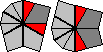Sometimes a layer is ambiguous, because it belongs to both cases 3 and 4 above. In particular, the layer shape CCECEEEEE can be split as EE-EE-C-C-ECE, or as C-EE-EE-ECCE. We will use this ambiguity later on.

So, the first thing to do to move towards the cube shape is to pair up the edge pieces. Getting three pairs is fairly easy, in fact, in more than half the configurations there are already three pairs. In nearly all other cases there will be unpaired edges in both layers. It is then possible to bring an unpaired edge in the top layer to the front to the left of the seam, and an unpaired edge in the bottom layer to the back to the right of the seam so that a single twist brings the two edges together and does not split any already paired edges. This process can be repeated if necessary until you have at least three edge pairings. There is one special case you should be aware of, where all unpaired edges are in a single layer which only occurs when it is a square shape. In this case simply do any twist (that does not split any pairs in the other layer) to spread the unpaired edges to both layers and then continue the process outlined above to bring them together.

You will now have at least 3 pairs of edges. There are now three possibilities left:

1. You already have 4 pairs of edges. It is now a simple matter to bring these pairs together to form a large group of 8 adjacent edges.
2.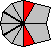You have two unpaired edges which are 150 degrees apart, i.e. they are separated by two corner pieces. Turn the layer with these two edges so that they both lie on the left hand side of the seam. I prefer to have them at the bottom left so I turn over the puzzle if they are on top. You next use only turns of the top layer and twists of the right hand side to move the three edge pairs together in a large group of 6 edges in the top layer. Often this is easy. The trickiest case is when the bottom right half consists of an edge pair between two corners. This pair cannot be immediately placed next to another edge pair, so you will have to twist it to the top and bring an edge pair to the bottom so that it lies next to the seam. Just be patient and make sure not to turn the bottom layer or to break up the edge pairs. Once you have the large group of 6 edges you can place them next to the two single edges to make a group of 8 edges together.
3.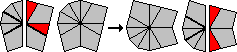You have two single edges that are 90 degrees apart, i.e. they are separated by exactly one corner piece. Turn the layer with these two edges so that they both lie on the same side of the seam, and turn the puzzle so that they are in the left half of the bottom layer. Using only turns of the top layer and twists of the right half of the puzzle bring (at least) two of the edge pairs together in the top layer to make a group of 4 (or 6) adjacent edges. It is now always possible to bring the single edges and the group of 4 together in one layer to make the layer shape CCECEEEEE (or its mirror image CCEEEEECE), and this leaves (or moves) the remaining edge pair to the other layer. Because of the ambiguity of the edge pairings in this shape, you can consider the two edges on either side of the two adjacent corners to be the unpaired ones instead. This can therefore be solved as in case 2 above.
[Note: You could use the ambiguity of the CEEECCEEE layer shape instead but this it is slower to solve because its edge pairs are separated, and you need to put all three edge pairs together in case 2.]

At this point all eight edges are together in one layer. A single simple sequence of moves now recovers the cube shape. Split the group of edges in two, making both layers into the scallop shape (CCCCEEEE). Now split each set of edges in two again to get both layers into the barrel shape (CCEECCEE). Split one barrel between a pair of corners and the other between a pair of edges, to get both layers into the kite shape (CECEECEC). If you split these between their edge pairs you form two squares.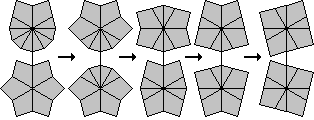The above should give you enough information to eventually get two squares. If the middle layer is not square, a single twist can make it so, though it is not yet necessary to do this at this point. The middle layer could well get disturbed in the later stages, but it can always be solved independently at the end.

## Section 2:

Solving the pieces.

## Notation:

From now on we need a notation for the moves. Hold the puzzle with the 'square 1' text on the left, so that the cut in the middle layer goes from the front left to the back right. I hold my left thumb on the small front-left face of the middle layer, and my left fingers on the back. I use my right hand to turn the top or bottom layers, as well as the right hand half of the puzzle.

Turns of the top and bottom layers are denoted by a pair of numbers (n,m). They are the multiple of 30 degrees that the top/bottom layers are to turn respectively. A 3 means clockwise 90 degrees, 1 means 30 degrees (i.e. one edge along) and negative numbers mean anti-clockwise turns. A twist of the right hand side of the puzzle is denoted by a /. As the puzzle is in the cube shape now, the move sequences (1,0)/(-1,0) or (0,-1)/(0,-1) for example both leave the puzzle in cube shape if you ignore the middle layer.

As a shorthand, I will also use letters to denote the possible positions of the pieces in the cube. This notation is based on that of the Rubik's Cube. Let the faces be denoted by L, R, F, B, U and D (left, right, front, back, up, down). Positions of edge pieces can be denoted by two letters, for example the edge position on the left of the top layer is denoted UL. Similarly positions of corners are denoted by 3 letters, for example DFR is the front-right corner position of the bottom layer.

## A short note about odd permutations:

Now that the puzzle is a cube shape, it is tempting to think that it can now be solved without changing shape. Many of the sequences used below indeed keep the top and bottom layers square. Each shape preserving move swaps two pairs of corners and two pairs of edges. This is an even number of swaps, so this is an even permutation. No combinations of even permutations can possibly be an odd permutation so any position that needs an odd number of swaps to solve it, cannot be solved without changing shape. In particular, to make a single swap of pieces you must change the shape of the puzzle. The quickest way to perform an odd permutation is to go to the scallop-scallop shape (3 twists), swap three corners from one layer with three from the other (1 twist), and return to the cube shape (3 twists) which takes 7 twists in total.

There are several methods for placing the pieces into their correct positions. I will show the most common ones here.

Method 1:
This first method was worked out and given to me by Bobfish. The pieces are first put into their own layers, then the corners are placed, and finally the edges. Some of the sequences used in this solution can be made slightly shorter. If you want, you can look them up in the computer generated tables that are listed in method 2 below. I have left this solution as is, because Bobfish found these sequences all by hand without computer. The parity fixer in particular is especially impressive - it is a much better clean odd edge swap than I ever found by hand myself.

Phase 1: Put pieces in their layers.
This phase puts all the white pieces in the top layer, and all the green pieces in the bottom layer. You can always keep the top an bottom layers square throughout this phase.

1. First put all the green corners in the bottom layer. This is quite easy if you first put two green ones in the left half of the bottom layer, then pair up the other two green corners and also twist them into the bottom layer.
2. You can now make the middle layer square if necessary by doing the move sequence /(6,0)/(6,0)/(6,0). All move sequences later on will have an even number of twists, so will leave the middle layer square.
3. Now bring the edges to their layer. There are 8 possible cases, which are listed below. In each case I will list the positions where there are edges in the wrong layer.
1. All edges are in the correct layer: do nothing.
2. UB, DL: do moves (1,0)/(-3,0)/(-1,-1)/(3,0)/(1,-2)/(-3,0)/(-1,-1)/(4,1)/(-1,0)
3. UF, UB, DF, DB: do moves (1,0)/(-1,-1)/(0,1).
4. UB, UL, DR, DB: do moves (1,0)/(-3,0)/(-1,-1)/(4,1)/(-1,0)
5. UB, UL, DF, DB: do moves (1,0)/(3,0)/(-1,-1)/(-3,0)/(4,1)/(-3,0)/(-1,-1)/(4,1)/(-1,0)
6. UF, UB, DB, DL: do moves (0,-1)/(-3,0)/(1,1)/(3,0)/(0,-3)/(-3,0)/(-1,-1)/(4,1)/(-1,0)
7. UF, UR, UB, DF, DB, DL: do moves (1,0)/(-1,-1)/(-2,1)/(-3,0)/(-1,-1)/(3,0)/(1,-2)/(-3,0)/(-1,-1)/(4,1)/(-1,0), or apply sequence 3 and then sequence 2.
8. All edges wrong: do moves (1,0)/(-1,-1)/(-2,-2)/(-1,-1)/(0,1), or apply sequence 3 twice.

Phase 2: Put the corners correct.
This phase puts all the corners correct, while leaving the all edges in their own layers.

1. This phase can be done using only two move sequences:
1. Swap UFR-UBL, DFR-DBR: /(3,0)/(-3,0)/(3,0)/(3,0)/(6,0)/
2. Swap UFR-UBR, DFL-DBR: /(0,-3)/(0,3)/(0,-3)/(0,-3)/(0,6)/
If you want to keep the layers square, you may do (1,0) before performing these, and (-1,0) at the end.
2. The second sequence is actually the same as the first performed upside down.
3. You will probably have to apply these sequences more than once before the corners are solved.
4. If you only need a single corner swap, then give that layer a quarter turn. Its corners now need a three-cycle, which can be done by twice swapping adjacent corners.

Phase 3: Put the edges correct.

1. Most of this phase can be done using only two move sequences:
1. Swap UF-UB, DF-DB: (1,0)/(-1,-1)/(6,0)/(1,1)/(-1,0)
2. Swap UL-UF, DR-DF: (1,0)/(-3,0)/(-1,-1)/(3,0)/(1,1)/(-3,0)/(-1,-1)/(4,1)/(-1,0)
2. You will probably have to apply these sequences more than once before the edges are mostly solved.
3. These sequences all perform two swaps. It is possible that you need an odd number of swaps to solve the edges. To this end you will have to fix the parity by using the following move sequence:
3. Swap UF-UB: /(3,3)/(1,0)/(-2,-2)/(2,0)/(2,2)/(-1,0)/(-3,-3)/(-2,0)/(3,3)/(3,0)/(-1,-1)/(-3,0)/(1,1)/(-4,-3)

Method 2:
This method solves the corners completely, then the edges in their layers, and finally the edges completely.

Phase 1: The corner pieces.
In this phase, every twist will keep the layers square.

1. Find the piece that should be at UFL. If it is in the bottom layer, then bring it to the top with a twist, (keeping the U,D layers square by turning either layer slightly before twisting). Then turn the top to place it correctly.
2. Find the piece that should be at UBL. If it is incorrectly placed in the top layer, then bring it to the bottom with a twist. Then turn the bottom to place it at DFR. Now do (4,0)/(-4,0)
3. Find the piece that should be at UBR. If it is incorrectly placed in the top layer, then bring it to the bottom with a twist. Then turn the bottom to place it at DFR. Now do the twist (1,0)/(-1,0).
4. Find the piece that should be at UFR. If it is not in place then turn the bottom to place it at DFR. Now do the sequence (1,0)/(0,-3)/(0,3)/(-1,0).
5. Rotate the bottom layer to place at least 2 corners are correct. If you need to swap two corners, then you can use the following two sequences:
1. Swap DFR-DBR: (1,0)/(0,-3)/(3,0)/(-3,0)/(-3,3)/(-3,0)/(2,0)
2. Swap DFL-DBR: (1,0)/(3,3)/(0,3)/(3,3)/(0,3)/(3,3)/(-1,-3)
It is possible to perform the second (diagonal) swap by using the first (adjacent) swap twice.

Phase 2: Place the edge pieces in their correct layer.
Rotate the top/bottom layers to bring it to a position where one of the following sequences will place the edges in the correct layer. I have tried to include as many useful cases as possible in this table, but there is no need to memorise all of these. If you know only one of the sequences 8 to 13, you can use it repeatedly until the edges are in the correct layer, though using sequence 3 and 4 will speed things up.

 1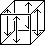Swap DF-UF, DR-UR, DB-UB, DL-UL (3,2)/(1,1)/(-3,3)/(-1,-1)/(0,-5) [4*|13*] 2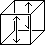Swap DF-UF, DB-UB (0,5)/(1,1)/(-1,6) [2*|7*] 3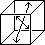Swap DF-UB, DB-UF (0,-1)/(1,1)/(-1,0) [2*|6*] 4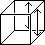Swap DR-UR, DB-UB (0,2)/(0,3)/(1,1)/(-1,-4)/(0,-2) [4*|11*] 5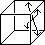Swap DR-UB, DB-UR (0,2)/(0,3)/(1,-5)/(-1,5)/(0,3)/(0,-2) /(0,3)/(0,-4)/(1,1)/(-1,3)/(0,-3)/ [5*|13*][6|13*] 6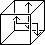Swap DB-UB, DR-UF /(-3,0)/(0,5)/(6,1)/(0,3)/(-5,0)/(-1,6) [6*|14*] 7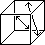Swap DB-UF, DR-UB (1,0)/(0,5)/(0,-3)/(-1,6)/(0,-5)/(0,3)/(0,6) [6*|14*] 8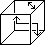Swap DR-UF, UR-UB (1,0)/(0,-3)/(-1,2)/(1,1)/(0,-3)/(0,3)/(-1,0) [6*|15*] 9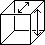Swap DR-UR, UF-UB (1,0)/(0,-3)/(-1,-4)/(1,1)/(0,3)/(0,3)/(-1,0) [6*|15*] 10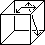Swap DR-UB, UF-UR (0,-1)/(0,3)/(0,-3)/(1,1)/(-1,2)/(0,-3)/(0,1) [6*|15*] 11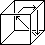Cycle UF->UR->DR (-5,-3)/(5,0)/(0,3)/(-5,0)/(5,0)/(0,-3)/(0,3) [6*|14*] 12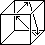Cycle UF->UB->DR (1,0)/(-1,0)/(0,3)/(-5,0)/(5,0)/(0,-3)/(1,0)/(5,0) [7*|15*] 13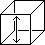Swap UF-DF (0,-3)/(0,3)/(1,0)/(2,-2)/(4,0)/(4,0)/(-4,4)/(0,1)/(0,3)/(-3,-1)/(1,-2)/(-1,0) [11*|27*]

Phase 3: Place the edge pieces in each layer correctly.
Rotate the top/bottom layers to bring it to a position where one of the following sequences will place the edges correctly. There is no need to memorise all of the sequences, as only a few of the single layer swaps will be sufficient. Alternatively any odd permutation together with sequences a4 and a5 will do.

1. The following sequences perform swaps in both layers:
 1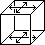Swap UF-UB, UR-UL, DF-DB, DR-DL (1,0)/(2,-4)/(1,1)/(2,2)/(-5,1)/(5,0) [5*|15*] 2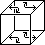Swap UF-UL, UR-UB, DF-DL, DR-DB (0,2)/(-3,0)/(1,1)/(3,-3)/(-1,-1)/(0,3)/(0,-2) [6*|16*] 3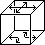Swap UF-UB, UR-UL, DF-DL, DR-DB /(3,3)/(0,3)/(1,-5)/(4,-2)/(4,1)/(3,3)/ [7*|18*] 4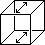Swap UF-UB, DF-DB (1,0)/(5,-1)/(-5,1)/(5,0) [3*|9*] 5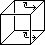Swap UR-UB, DR-DB (0,2)/(0,-3)/(1,1)/(-1,2)/(0,-2) [4*|11*] 6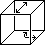Swap UF-UB, DR-DB (1,0)/(0,-1)/(0,-3)/(5,0)/(-5,0)/(0,3)/(0,1)/(5,0) [7*|15*] 7Swap UF-UB, UL-UR, DF-DB /(3,3)/(0,-3)/(3,3)/(0,-2)/(-2,4)/(2,-4)/(0,-1)/(3,3)/(0,3) [9*|23*]
2. The following sequences move top layer edges only:
 1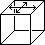Swap UF-UB, UR-UL /(3,-3)/(3,-3)/(0,1)/(-3,3)/(-3,3)/(-1,0) [6*|16*] 2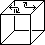Swap UF-UL, UR-UB /(3,3)/(0,3)/(1,1)/(-1,-4)/(-3,-3)/ [6*|15*] 3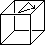Cycle UF->UB->UR (1,0)/(-1,2)/(-5,1)/(0,3)/(-3,0)/(5,2)/(-5,4)/(-4,0) (-3,0)/(3,0)/(1,0)/(0,-3)/(-1,0)/(-3,0)/(1,0)/(0,3)/(2,0) [7*|19][8|17*] 4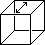Swap UF-UB (3,0)/(3,3)/(-1,0)/(2,-4)/(4,-2)/(0,-2)/(-4,2)/(1,-5)/(3,0)/(3,3)/ [10*|26*] 5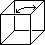Swap UF-UR (0,3)/(-3,-3)/(0,-5)/(-2,2)/(0,4)/(0,-4)/(-1,2)/(3,0)/(3,4)/(0,-4)/(0,1)/(3,0)/ (0,3)/(-3,0)/(0,3)/(0,-3)/(0,3)/(2,0)/(0,2)/(-2,0)/(4,0)/(0,-2)/(0,2)/(-1,4)/(0,-3)/ [12*|28][13|27*] 6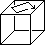Cycle UF->UL->UB->UR (1,0)/(2,2)/(0,-2)/(3,3)/(1,0)/(4,4)/(0,-2)/(2,2)/(0,-1)/(3,3)/ [10*|25*] 7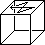Cycle UB->UF->UR->UL (-3,2)/(1,-2)/(-4,0)/(0,3)/(1,0)/(3,-2)/(-4,0)/(-4,0)/(-2,2)/(-1,0)/(0,-3)/ [11*|26*]

Phase 4: Fix the central layer.

1. If the middle layer is not a square, then the following sequence will correct it by reversing its right hand side:
/(6,0)/(6,0)/(6,0)

Method 3: Edges First.
First solve the edges completely, in the same way as phase 1 of method 2. Then put the corners in their layers and solve them using the sequences in the tables below:

1. The following move corners from one layer to the other.
1. Swap UFR-DFR, UBR-DBR, UBL-DBL, UFL-DFL: (4,0)/(2,2)/(-3,3)/(-2,-2)/(-1,-3)   [4*|13*]
2. Swap UFL-DFL, UBR-DBR: (4,0)/(2,2)/(6,-2)   [2*|7*]
3. Swap UFL-DBR, UBR-DFL: (0,2)/(-2,-2)/(2,0)   [2*|6*]
4. Swap UFL-DFL, UFR-DFR: (1,0)/(3,0)/(2,2)/(-5,-2)/(-1,0)   [4*|11*]
5. Swap UFL-DFR, UFR-DFL: (1,0)/(3,0)/(-4,2)/(4,-2)/(3,0)/(-1,0)   [5*|13*], or /(3,0)/(-5,0)/(2,2)/(3,-2)/(-3,0)/   [6|13*]
6. Swap UFL-DFL, UBR-DFR: /(3,0)/(0,-4)/(6,-2)/(0,-3)/(4,0)/(2,6)   [6*|14*]
7. Swap UFL-DFR, UBR-DFL: (0,6)/(0,3)/(0,-4)/(4,0)/(-3,0)/(6,-2)/(2,0)   [6*|14*]
8. Swap UFR-UBR, UFL-DFR: (0,2)/(0,-3)/(-2,1)/(2,2)/(0,-3)/(0,3)/(0,-2)   [6*|15*]
9. Swap UFL-UBR, UFR-DFR: (0,2)/(0,-3)/(-2,-5)/(2,2)/(0,3)/(0,3)/(0,-2)   [6*|15*]
10. Swap UFL-UFR, UBR-DFR: (-2,0)/(0,3)/(0,-3)/(2,2)/(-2,1)/(0,-3)/(2,0)   [6*|15*]
11. Cycle UFL->UFR->DFR: (4,5)/(3,0)/(-4,0)/(0,4)/(0,-3)/(0,-4)/(0,-5)   [6*|14*]
12. Cycle UFL->UBR->DFR: (-2,0)/(2,0)/(0,-3)/(4,0)/(-4,0)/(0,3)/(-2,0)/(-4,0)   [7*|15*]
13. Swap UFR-DFR: /(3,3)/(-1,0)/(2,-2)/(0,-1)/(0,3)/(-2,-1)/(2,0)/(-1,-2)/(-3,0)/   [10*|23*]
2. The following move corners within both layers.
1. Swap UFR-UBL, UFL-UBR, DFR-DBL, DFL-DBR: (1,0)/(-1,5)/(3,3)/(1,1)/(-3,3)/(5,0)   [5*|15*]
2. Swap UFR-UFL, UBL-UBR, DFR-DFL, DBL-DBR: (1,0)/(0,-3)/(2,2)/(-3,3)/(-2,-2)/(3,0)/(-1,0)   [6*|16*]
3. Swap UFL-UBR, DFL-DBR: (0,5)/(-2,4)/(2,-4)/(0,1)   [3*|9*]
4. Swap UFL-UFR, DFL-DFR: (1,0)/(-3,0)/(2,2)/(1,-2)/(-1,0)   [4*|11*]
5. Swap UFL-UBR, DFL-DFR: (4,0)/(0,2)/(0,3)/(-4,0)/(4,0)/(0,-3)/(0,-2)/(2,0)   [7*|15*]
6. Swap UFL-UBR, UFR-UBL, DFL-DBR: /(3,3)/(1,2)/(2,-4)/(4,-2)/(-2,-1)/(3,3)/   [7*|19*]
3. The following move corners in the top layer only.
1. Swap UFL-UBR, UFR-UBL: /(3,-3)/(3,-3)/(0,-2)/(-3,3)/(-3,3)/(2,0)   [6*|16*]
2. Swap UFL-UFR, UBR-UBL: /(3,3)/(-5,-2)/(2,2)/(3,0)/(-3,-3)/   [6*|15*]
3. Cycle UFL->UBR->UFR: (1,0)/(0,3)/(2,0)/(-3,0)/(-2,0)/(0,-3)/(2,0)/(3,0)/(-3,0)   [8*|17*]
4. Swap UFL-UBR: /(3,3)/(3,0)/(-4,2)/(-2,4)/(6,2)/(-4,2)/(-2,4)/(-5,0)/(-3,-3)/   [10*|26], or /(-3,0)/(0,-3)/(2,0)/(0,2)/(-2,0)/(4,0)/(0,-2)/(0,2)/(-4,1)/(3,0)/   [11|22*]
5. Swap UFL-UFR: /(3,3)/(1,4)/(0,4)/(-2,4)/(-4,2)/(2,0)/(-2,4)/(4,1)/(3,3)/   [10*|26], or /(0,3)/(1,2)/(0,-4)/(2,-2)/(0,-4)/(0,-4)/(0,4)/(0,-4)/(-3,0)/(0,-3)/   [11|23*]
6. Cycle UFL->UBL->UBR->UFR: /(3,3)/(-1,0)/(-2,-2)/(2,0)/(2,2)/(0,-2)/(2,2)/(-1,0)/(-3,-3)/   [10*|24*]

Method 4: Layer method

Phase 1: Put pieces in their layers.
This phase puts all the white pieces in the top layer, and all the green pieces in the bottom layer. You can always keep the top an bottom layers square throughout this phase.

1. First put all the green corners in the bottom layer. This is quite easy if you first put two green ones in the left half of the bottom layer, then pair up the other two green corners and also twist them into the bottom layer.
2. Now bring the edges to their layer. There are 8 possible cases, which are listed below. In each case I will list the positions where there are edges in the wrong layer.
1. All edges are in the correct layer: do nothing.
2. UB, DF: do moves (0,-1)/(-3,0)/(4,1)/(-4,-1)/(3,0)/(0,1)   [5*|13], or (-2,0)/(5,0)/(0,3)/(-5,0)/(5,0)/(0,-3)/   [6|12*]
3. UF, UB, DF, DB: do moves (-1,0)/(1,1)/(0,-1)   [2*|4*]
4. UB, UR, DL, DB: do moves (1,0)/(0,3)/(-1,-1)/(0,-3)/(0,1)   [4*|10*]
5. UF, UR, DF, DB: do moves (1,0)/(0,-3)/(0,3)/(2,-1)/(-3,0)/(0,1)   [5*|12*]
6. UF, UB, DF, DR: do moves (0,-1)/(3,0)/(-3,0)/(1,-2)/(0,3)/(-1,0)   [5*|12*]
7. UR, UB, UL, DF, DR, DB: do moves (0,-1)/(1,4)/(-1,-4)/(-3,0)/(4,1)/(-1,0)   [5*|14*]
8. All edges wrong: do moves (-1,0)/(1,1)/(2,2)/(1,1)/(0,-1)   [4*|12*], or apply sequence 3 twice.

Phase 2: Solve each layer separately.
The following table shows all sequences that solve the top layer for each possible case, except for the ones already listed before, such as those in method 2, part 3b. The bottom layer can be solved in the same way using the mirror images of these sequences (or by turning over the puzzle first).

1. The following swap the diagonally opposite corners UFL and UBR, as well as the U layer edges as shown:
1. B-L: (4,0)/(3,0)/(3,3)/(3,0)/(5,0)/(0,3)/(3,3)/(0,3)/   [8*|18*]
2. B-R: (1,0)/(3,0)/(3,3)/(3,0)/(5,0)/(0,3)/(3,3)/(0,3)/(3,0)   [8*|19*]
3. L-R: (-3,0)/(3,3)/(3,0)/(3,3)/(3,0)/(3,3)/   [6*|15*]
4. F-B: (1,0)/(3,3)/(3,0)/(3,3)/(3,0)/(3,3)/(2,0)   [6*|16*]
5. R->B->F: (-2,-1)/(0,3)/(0,1)/(-4,0)/(4,0)/(4,0)/(0,4)/(-4,0)/(2,1)/(3,-3)/(3,0)/(-1,-2)   [11*|27*]
6. R->L->F: /(3,-3)/(0,-3)/(-1,4)/(-4,0)/(0,4)/(4,0)/(4,0)/(-4,0)/(0,1)/(0,3)/(0,6)   [11*|24*]
7. B-L, F-R: /(3,3)/(-1,4)/(4,-2)/(2,-4)/(0,2)/(4,-2)/(-5,1)/(3,0)/(3,3)/   [10*|26*]
8. L-F, B-R: /(3,3)/(0,3)/(5,-1)/(-4,2)/(-2,0)/(-2,4)/(-4,2)/(2,1)/(3,3)/   [10*|26*]
2. The following swap the adjacent corners UBL and UBR, as well as the U layer edges as shown:
9. R-F: (1,0)/(3,3)/(-4,-1)/(1,4)/(0,-3)/(0,-3)/(3,0)/(0,-3)/(-4,0)   [8*|20*]
10. B-R: /(-3,3)/(3,0)/(0,-3)/(0,3)/(0,-3)/(3,0)   [6*|13*]
11. L-B: (1,0)/(-3,3)/(3,0)/(0,-3)/(0,3)/(0,-3)/(2,0)   [6*|14*]
12. F-L: /(-3,-3)/(4,1)/(-4,-1)/(0,3)/(3,0)/(-3,0)/(3,0)/(3,0)   [8*|19*]
13. L-R: (0,-1)/(1,1)/(3,0)/(-3,0)/(-1,-4)/(3,0)/(-3,0)/(-2,4)/(3,0)/(2,0)   [9*|22*]
14. F-B: (3,0)/(3,0)/(3,3)/(3,0)/(4,0)/(0,3)/(3,3)/(0,3)/(2,0)   [8*|19*]
15. R->L->F: /(0,-3)/(-2,0)/(-3,-1)/(-1,2)/(0,-4)/(0,4)/(1,-4)/(0,3)/(0,-5)/(0,3)/(3,0)/(5,0)   [12|27*] or /(0,3)/(0,-3)/(-5,0)/(0,3)/(0,5)/(0,-4)/(4,0)/(-2,-2)/(0,2)/(0,1)/(3,0)/(0,-1)/(0,4)   [13|27*] or (3,3)/(1,6)/(-2,4)/(0,2)/(0,5)/(0,3)/(-2,-1)/(4,0)/(-4,0)/(3,-2)/(-3,0)/(0,6)   [11*|29]
16. B->F->R: /(3,0)/(1,2)/(2,0)/(-1,-2)/(0,3)/(-1,0)/(2,0)/(-2,0)/(-1,0)/(-3,-3)/   [11*|24*]
17. L->R->B: /(3,0)/(0,2)/(0,-3)/(3,0)/(2,0)/(0,4)/(0,4)/(-4,0)/(-1,0)/(-3,0)/(-3,-4)/(0,4)   [12|25*] or (1,-1)/(0,3)/(2,1)/(0,4)/(4,-4)/(-2,3)/(0,3)/(-2,3)/(0,-4)/(-2,0)/(-3,0)/(5,1)   [11*|29]
18. F->B->L: /(3,0)/(1,0)/(-2,0)/(0,5)/(0,-1)/(4,0)/(0,2)/(-4,1)/(0,1)/(0,5)/(-3,0)/(-1,1)   [12|26*] or (4,-1)/(0,3)/(-3,0)/(1,-1)/(4,0)/(2,-2)/(4,0)/(4,0)/(-4,0)/(1,0)/(0,3)/(5,-2)   [11*|27]
19. F-L, B-R: /(3,3)/(0,3)/(1,-1)/(-2,0)/(0,2)/(0,-4)/(2,0)/(-2,0)/(1,0)/(-3,-3)/   [11|24*] or (-3,-3)/(-3,-3)/(-4,5)/(-4,0)/(4,-2)/(-4,2)/(-2,0)/(5,1)/(-3,0)/(3,3)/   [10*|27]
20. F-R, B-L: /(3,3)/(-1,2)/(-4,2)/(4,0)/(-2,4)/(-4,2)/(-1,1)/(3,0)/(3,3)/   [10*|26*]
21. L-R, F-B: /(3,3)/(-1,0)/(2,0)/(0,4)/(0,-4)/(2,2)/(0,-4)/(2,0)/(0,1)/(3,3)/(6,0)   [11|25*] or /(3,3)/(1,-2)/(0,4)/(-2,4)/(2,-4)/(-1,1)/(5,-1)/(-2,1)/(3,3)/   [10*|27]
22. F->R->L->B: (-3,0)/(-3,0)/(3,3)/(3,0)/(1,-2)/(-1,2)/(-3,-3)/   [7*|18*]
23. F->L->R->B: (4,0)/(-3,0)/(3,3)/(3,0)/(2,-1)/(-2,1)/(-3,-3)/(-1,0)   [7*|19] or /(-3,3)/(3,-3)/(1,0)/(0,3)/(0,-3)/(0,3)/(0,-3)/(2,0)   [8|18*]

Method 5: Alternative layer method

Phase 1: Put pieces in their layers.
This phase is the same as in Method 4.

Phase 2: Adjust the permutation parities of the layers.
This phase make sure that each layer is in itself an even permutation. This cuts down the number of possibilities, leaving fewer (and generally shorter) sequences in the last phases. It is however slightly tricky to recognize the parities quickly.

1. Depending on the parities of the layer permutations, do one of the following:
1. Both layers even parity: Do nothing.
2. Top layer odd, bottom even: /(-3,-3)/(0,-1)/(2,-4)/(4,-2)/(1,0)/(-3,-3)/
3. Top layer even, bottom odd: /(3,3)/(1,0)/(4,-2)/(2,-4)/(0,-1)/(3,3)/
4. Both layers odd parity: (1,0)/(5,-1)/(-5,1)/(-1,0)

Phase 3: Solve the first layer.
The following table shows the sequences that solve the top layer for each possible case. Note that these sequences may mix up the (unsolved) bottom layer.

1. The following sequences move only corners:
 1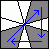Cycle UFL->UBR->UFR, (-2,0)/(0,-3)/(2,2)/(0,-3)/(-2,4)/(2,0) [5|13*] 1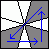Cycle UFL->UFR->UBR, (-2,0)/(2,-4)/(0,3)/(-2,-2)/(0,3)/(2,0) [5|13*] 1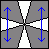Swap UFL-UBL, UFR-UBR /(3,3)/(1,-2)/(2,2)/(-3,0)/(-3,-3)/ [6|15*]
2. The following sequences move only edges:
 1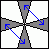Swap UL-UF, UR-UB /(3,3)/(0,3)/(1,1)/(-1,-4)/(-3,-3)/ [6|15*] 2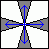Swap UL-UR, UF-UB (1,0)/(3,-3)/(2,2)/(3,3)/(4,-2)/(-1,0) [5|15*] 3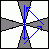Cycle UF->UB->UR (0,-1)/(0,-3)/(1,1)/(0,-3)/(-1,5)/(0,1) [5|13*] 4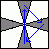Cycle UF->UR->UB (0,-1)/(1,-5)/(0,3)/(-1,-1)/(0,3)/(0,1) [5|13*]
3. The following sequences swaps the UFL,UBL corners and moves edges:
 1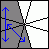Swap UFL-UBL, UL-UF (-2,0)/(0,3)/(-3,-3)/(3,0)/(2,0) [4|10*] 2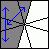Swap UFL-UBL, UL-UB (3,0)/(3,0)/(3,3)/(3,0)/(3,0) [4|10*] 3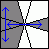Swap UFL-UBL, UL-UR (-2,0)/(-3,0)/(2,2)/(0,3)/(1,-5)/(2,0) [5|13*] 4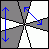Swap UFL-UBL, UB-UR (1,0)/(3,3)/(2,-1)/(-2,1)/(-3,-3)/(-1,0) [5|15*] 5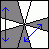Swap UFL-UBL, UR-UF /(3,3)/(1,-2)/(-4,5)/(3,3)/ [5|13*] 6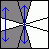Swap UFL-UBL, UB-UF (1,0)/(2,-1)/(1,-2)/(2,-1)/(-5,4)/(5,0) [5|15*] 7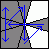Swap UFL-UBL, Cycle UL->UB->UF->UR (-2,0)/(0,-3)/(3,3)/(2,-1)/(3,3)/(4,-2)/(-1,0) [6|17*] 8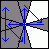Swap UFL-UBL, Cycle UB->UR->UL->UF /(3,3)/(1,4)/(2,-4)/(3,0)/(-3,-3)/ [6|15*] 9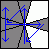Swap UFL-UBL, Cycle UR->UF->UB->UL (-2,0)/(3,3)/(3,0)/(5,-1)/(1,-2)/(3,3)/(2,0) [6|17*] 10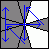Swap UFL-UBL, Cycle UF->UL->UR->UB /(3,3)/(0,3)/(1,-5)/(-4,-1)/(3,3)/ [6|15*]
4. The following sequences swaps the UFL,UBR corners and moves edges:
 1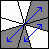Swap UFL-UBR, UR-UF (-2,0)/(2,-1)/(4,-5)/(-1,2)/(4,-5)/(2,0) [5|15*] 2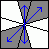Swap UFL-UBR, UB-UF (1,0)/(-3,3)/(3,-3)/(-1,0) [3|9*] 3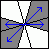Swap UFL-UBR, UL-UR /(-3,3)/(3,-3)/ [3|7*] 4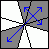Swap UFL-UBR, UB-UR (-2,0)/(2,-4)/(0,3)/(1,1)/(-3,0)/(2,0) [5|13*]

Phase 4: Solve the second layer.
Turn the puzzle over, and solve the top layer using the sequences in the table below. This table is very similar to the one in the previous phase, except that the move sequences are generally a bit longer, because these have to keep the bottom layer intact.

1. The following sequences move only corners:
 1Cycle UFL->UBR->UFR, (1,0)/(0,3)/(2,0)/(-3,0)/(-2,0)/(0,-3)/(2,0)/(3,0)/(-3,0) (1,0)/(-1,2)/(-5,-2)/(-3,0)/(3,0)/(-4,2)/(1,-2)/(-4,0) [8|17*] [7*|19] 2Cycle UFL->UFR->UBR, (3,0)/(-3,0)/(-2,0)/(0,3)/(2,0)/(3,0)/(-2,0)/(0,-3)/(-1,0) (-2,0)/(2,-1)/(-2,4)/(-3,0)/(3,0)/(-1,-4)/(-2,1)/(5,0) [8|17*] [7*|19] 3Swap UFL-UBL, UFR-UBR /(3,3)/(1,-2)/(2,2)/(-3,0)/(-3,-3)/ [6*|15*]
2. The following sequences move only edges:
 1Swap UL-UF, UR-UB /(3,3)/(0,3)/(1,1)/(-1,-4)/(-3,-3)/ [6*|15*] 2Swap UL-UR, UF-UB /(3,-3)/(3,-3)/(0,1)/(-3,3)/(-3,3)/(-1,0) [6*|16*] 4Cycle UF->UR->UB (-2,0)/(0,-3)/(-1,0)/(3,0)/(1,0)/(0,3)/(-1,0)/(-3,0)/(3,0) (-2,0)/(2,-1)/(4,1)/(3,0)/(-3,0)/(5,-1)/(-2,1)/(5,0) [8|17*] [7*|19] 3Cycle UF->UB->UR (-3,0)/(3,0)/(1,0)/(0,-3)/(-1,0)/(-3,0)/(1,0)/(0,3)/(2,0) (1,0)/(-1,2)/(1,-5)/(3,0)/(-3,0)/(2,5)/(1,-2)/(-4,0) [8|17*] [7*|19]
3. The following sequences swaps the UFL,UBL corners and moves edges:
 1Swap UFL-UBL, UL-UF (1,0)/(0,-3)/(0,3)/(0,-3)/(3,0)/(-3,3)/(2,0) [6*|14*] 2Swap UFL-UBL, UL-UB (3,0)/(-3,3)/(3,0)/(0,-3)/(0,3)/(0,-3)/ [6*|13*] 3Swap UFL-UBL, UL-UR /(0,3)/(3,3)/(0,3)/(4,0)/(3,0)/(3,3)/(3,0)/(5,0) [8*|18*] 4Swap UFL-UBL, UB-UR (1,0)/(3,0)/(-3,0)/(3,0)/(0,3)/(2,5)/(1,4)/(3,3)/(2,0) [8*|20*] 5Swap UFL-UBL, UR-UF /(3,3)/(1,-2)/(-4,-1)/(0,3)/(3,0)/(-3,0)/(3,0)/(0,-3) [8*|19*] 6Swap UFL-UBL, UB-UF (-2,0)/(-1,-1)/(-3,0)/(3,0)/(1,4)/(-3,0)/(3,0)/(2,-4)/(-3,0)/(0,1) [9*|22*] 7Swap UFL-UBL, Cycle UL->UB->UF->UR (1,0)/(0,3)/(0,-3)/(0,3)/(0,-3)/(2,0)/(-3,3)/(3,-3)/ (-2,0)/(3,3)/(2,-1)/(1,-2)/(0,3)/(-3,-3)/(-3,0)/(-1,0) [8|18*] [7*|19] 8Swap UFL-UBL, Cycle UB->UR->UL->UF /(3,3)/(1,4)/(2,-1)/(3,0)/(-3,-3)/(0,-3)/(0,-3) [7*|18*] 9Swap UFL-UBL, Cycle UR->UF->UB->UL /(-3,3)/(3,-3)/(-2,0)/(0,3)/(0,-3)/(0,3)/(0,-3)/(-1,0) (1,0)/(3,0)/(3,3)/(0,-3)/(-1,2)/(-2,1)/(-3,-3)/(2,0) [8|18*] [7*|19] 10Swap UFL-UBL, Cycle UF->UL->UR->UB /(-3,0)/(3,3)/(3,0)/(1,-2)/(-1,2)/(-3,-3)/(-3,0) [7*|18*]
4. The following sequences swaps the UFL,UBR corners and moves edges:
 1Swap UFL-UBR, UR-UF (1,0)/(2,2)/(3,0)/(0,-3)/(4,1)/(3,0)/(-3,0)/(5,-1)/(0,3)/(0,-2) [9*|22*] 2Swap UFL-UBR, UB-UF (1,0)/(3,3)/(3,0)/(3,3)/(3,0)/(3,3)/(2,0) [6*|16*] 3Swap UFL-UBR, UL-UR /(3,3)/(3,0)/(3,3)/(3,0)/(3,3)/(3,0) [6*|15*] 4Swap UFL-UBR, UB-UR (1,0)/(3,0)/(3,3)/(3,0)/(5,0)/(0,3)/(3,3)/(0,3)/(3,0) [8*|19*]

If you find that you have erred, and have a last layer with an odd permutation parity, the following move sequence will fix it while keeping the bottom layer intact.
/(-3,0)/(0,-3)/(2,0)/(0,2)/(-2,0)/(4,0)/(0,-2)/(0,2)/(-4,1)/(3,0)/    [11|22*]

Method 6: Pair up corners and edges

This is the solution I now use myself because it is so easy to remember. It essentially only uses one simple move sequence, plus any parity fixing sequence.

Phase 1: Pair up corners and edges.
This phase pairs up each corner with a matching edge so that the layers consist of four such corner/edge pairs.

Each corner forms a pair with the edge that lies adjacent to it in an anti-clockwise direction. So the UFL corner forms a pair with the UF edge, the DFR corner with the DF edge, and so on. At the moment most of these pairs do not have matching colours, but by the end of this phase they should.

Note that you can easily move pairs about without splitting them up by doing (1,0), followed by any combination of quarter turns (0,3) and (3,0), and twists /, and then ending with (-1,0) to return to the cube shape.

1. Find any unmatched pair. Look at the corner in that pair, and find the unmatched pair containing the edge that belongs with it.
2. Do an initial (1,0) move.
3. Do any combination of quarter turns and twists to put the pair with the corner in the top layer at the UFL location, and the pair with the edge in the bottom layer at the DBR/DR locations. Note that the top layer remains (1,0) out of alignment throughout this and the next steps.
4. Do /(3,0) which isolates the two mismatched pairs in the left half of the top layer.
5. If you can find a third other unmatched pair, then put this at the UFR location without moving the other two pairs. Then do (-4,0) followed by /(0,-3)/(0,3)/(0,-3)/(0,3)/, and then (1,0) again to put the layer out of alignment.
6. Repeat steps a-f until all pairs are matched.
7. If you cannot find a third other unmatched pair, then you will need to apply a parity fix. Any parity fixing sequence will do, but the following sequence keeps all the other corner/edge pairs intact. Starting with the top layer out of alignment, and the two mismatched pairs at the left of the top layer, do:
(3,0)/(0,3)/(0,-1)/(3,3)/(0,-5)/(-2,4)/(2,-4)/(0,-1)/(3,3)/
If you use any other parity fixing sequence you will probably have to go back to step a to match up the corner-edge pairs again.

Phase 2: Solve the outer layers.
The corner-edge pairs will now remain intact. This phase is equivalent to solving the corners of a Rubik's Domino.

1. Start with the top layer (1,0) out of alignment, so that twists will not split up the corner-edge pairs.
2. Do any combination of quarter turns and twists to put the correct pair at the DBL/DB location.
3. Find the pair that belongs at the DFL/DL location. If this pair is not yet in its solved position, then bring it to the UFR location and do (0,3)/(0,-3) to slot it into place.
4. Find the pair that belongs at the DBR/DR location. If this pair is not yet in its solved position, then bring it to the UFR location and do a / twist to slot it in place.
5. Find the pair that belongs at the DFR/DF location. If this pair is not yet in its solved position, then bring it to the UFR location and do a /(3,0)/(-3,0)/ to put it in place.
6. Examine the top layer pairs. If they are not yet solved relative to each other, then it must be possible to solve them by a swap of adjacent pairs or a swap of diagonally opposite pairs.
7. To swap two adjacent pairs, put them on the front of the top layer (still a little out of alignment) and do this: /(0,-3)/(0,3)/(0,-3)/(0,3)/, followed by (3,0), and then /(0,-3)/(0,3)/(0,-3)/(0,3)/ again.
8. To swap two diagonally opposite pairs, do (with the top layer still a little out of alignment): /(0,-3)/(0,3)/(0,-3)/(0,3)/, followed by (6,0), and then /(0,-3)/(0,3)/(0,-3)/(0,3)/ again.
9. Now turn the top layer so that it is correct.

Phase 3: Fix the central layer.

1. If the middle layer is not a square, then the following sequence will correct it by reversing its right hand side:
/(6,0)/(6,0)/(6,0)

Odds and Ends:

1. To swap top and bottom layers, do /(6,6)/(-1,1)   [4*|6*]
2. To change the middle layer shape, do /(0,6)/(0,6)/(0,6)   [3*|6*]